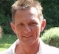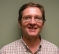## Polyphase Filter Bank channelizer issueStarted by 2 years ago5 replieslatest reply 2 years ago622 views

Hello, I am currently studying the implementation of a Polyphase Filter Bank channelizer designed by Xilinx in its XAPP1161. The architecture is composed by a single IP FIR handling eight different channels and an FFT module (plus some FIFOs). During the simulation phase I realized that the FIR is configured ("Sample Period" option of the IP GUI) to accept a sample every 89 clock cycles, which shall correspond to the total number of taps (712) divided by the number of channels (8).

Although I'm not very comfortable with the PFB channelizer theory, I can't understand why the FIR needs 89 cycles before to be ready to accept a new sample. Is there a special theoretical reason that explains this behavior or is this due to the internal architecture of the IP and how the latter manages the multiple channels?

From what I understand, if I use 8 separate FIRs I could provide each of them one sample every eight clock cycles, thus reaching 10 times the data throughput of the XAPP1161 implementation. Is this correct?

[ - ]Hello, I have worked on a PFB (Polyphase Filter Bank) channelizer ~6 months ago. I read the XAPP1161 and used the accompanying matlab code, but not the FPGA implementation, because I only had to do matlab + C implementation. I also forgot a lot since I quit that job :-) So I hope my answer can help.

"From what I understand, if I use 8 separate FIRs I could provide each of them one sample every eight clock cycles, thus reaching 10 times the data throughput of the XAPP1161 implementation. Is this correct?"

Yes, you have 8 filters of 89 taps (PFB rearrangement of a the 172 taps FIR prototype lowpass filter). Each accepting (and hopefully processing) one sample every 8 input samples. So each filter needs to process 1 sample every 8 clock cycles. But this is the sampling clock of the signal not necessary the one of the FPGA Filter, unless the 89 taps are calculated at once. Are you sure the Filter implementation is fully parallel and is not sharing part of the hardware that calculate one tap ? because in this case you would need the 89 FPGA Filter cycles, before accepting a new sample (that is coming every 8*Ts seconds, Ts=1/fs, Fs being the sampling frequency of the signal).

Regards,

Sara

[ - ]Hi. I have no insight in the Xilinx modules, however 89 clock cycles between the samples is no real challenge for the FPGA solution, since you would not clock it with 1.6MHz but certainly with something above 100MHz. 89*1.6MHz is ~142MHz.
The mentioned Virtex device would love to run with at least this rate.
So probably there is simply no need to make the design faster.
Especially, since this is for demonstration purposes and the intention is certainly to keep it simple, not perfected.

I'd assume that the Xilinx cores can be trimmed to more speed by trading it against resource usage or by reducing signal path width.
Usually you achieve that by telling the Core Generator the clock and signal rate requirements and it will try to find an implementation. If you allow it to run on a very slow signal rate, it will certainly try to do things sequentially resulting in a small resource foot print. If you require a high signal rate, work would be parallelized and the resource foot print would increase.

Use the CoreGenerator to reconfigure the core, test with different clock/rate-settings and compare the results. This might help you to understand what's going on.

(If you happen to use Xilinx System Generator, the generated code might depend on the Matlab settings, too.)

[ - ]Without knowing what the logic is doing it is hard to tell. Slowing each input 89 clocks demands buffering for all eight channels but it becomes guess work. Do you see simulation example or model example. You can model the FIR & fft in matlab and see how it is meant to work. My guess is that you need delay input by (1:8) plus any wait for zero insertion if upsampling (prior to any decimation for fractional sample rate change) yet 89/8 doesn't suggest that. The fft is frame based and may have its wait limitations added.

[ - ]I looked into theory of the Tx channeliser design and here is my initial understanding:

I assume our given inputs are some (n) baseband channels to be transmitted as one signal separated in frequency(FDM).

classic method: i) filter then ii) mix each channel to a frequency centre then iii) add all & iv)upsample/downsample to take account of sampling rate needed for the very edge after mixing.

fft based: one up/down polyphase filter can do same. Part of its internal computation happened to be same as fft, apparently as each complex filter is moved to new frequency centre. Thus fft completes the filter job and the context of fft here is not really matter of frequency/time domain conversion but just do the computations.

The math derivation is all around the web and it always mystifies concepts on me so I leave it for the math gurus.

[ - ]Hello,

I've done a couple of receive channelizers now.  I didn't use any canned tools.  I dug up several good papers and some Matlab example scripts.  I coded the FIR filters in Verilog by hand and verified them with Matlab and C generated vectors.

The design procedure for the FIR:  Given the number of channels, design a low pass filter that is an integer multiple of the channanizer in length.  Of course the FIR total length is determined by what is needed to meet your pass/stop band requirements.  So say if you have an 8 channel design you could wind up with a 4*8= 32 tap FIR.  If so then you will need to perform 4*2 multiplies for each incoming data sample assuming the samples are complex.  The number of clocks that takes per sample all depends on how you pipeline the filter.

For my design I have 2 clocks for every input sample.  Therefore I can re-use the same multipliers for both I and Q.  The bigger trick are the samples.  I need four samples: the current input sample and 3 previous samples that are N samples back. In our example that's 8, 16, and 32 samples ago.  So that means for every 2 clock cycles I have to write one sample and read three.  The output from the memory block is registered and presented 4 wide to the multiplier block.

I also need 4 tap weights.  I can look them up in parallel from a table based on the input count, 0 to 7.

The 8 point FFT also must be pipelined so that every 2 clock cycles the data advances one sample.

Hope this example is of some help.

Mark Napier